# Dirac monopole

(diff) ← Older revision | Latest revision (diff) | Newer revision → (diff)

A solution to the Maxwell equations describing a point source of a magnetic field. In 1931, P.A.M. Dirac [a1] considered the quantum mechanics of the electron in a magnetic field (due to a point source),(a1)

where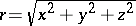is the length of the position vector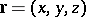in the Cartesian coordinates andis a constant determining the strength of the field, known as a magnetic charge of the monopole. Since the induction vector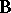in (a1) is central, it can be conveniently written in the spherical coordinatesdefined by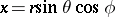,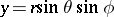,,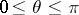,. In these coordinates, only the radial component ofis non-zero and equals. Maxwell's equations imply that there is no single vector potential corresponding todefined on the whole of. However, Dirac found that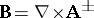, with vector potentials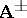whose only non-zero components are in the azimuthal direction and read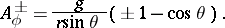(a2)

The potentials,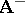are singular at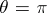(the negative-axis) and(the positive-axis), respectively. These singularities are known as Dirac's string singularities. The union of the regions in whichare well-defined covers the whole of. In the intersection of these regions (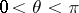) the vector potentialsare related by the gauge transformation,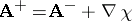, with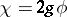. If there is an electron in the magnetic field, then in the region where bothandare well-defined, the wave functions of the electron corresponding to different vector potentials should be related by the gauge transformation, i.e.,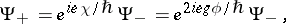whereis the electric charge of the electron and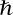is the Planck constant divided by. The wave function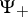is single valued if and only if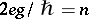for an integer, i.e. if and only if the magnetic charge attains discrete values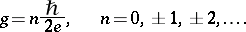(a3)

Thus, the consistency of the monopole field (a1) with quantum mechanics can be achieved, provided the magnetic chargebe quantized. Equation (a3) expresses also "duality" (reciprocity) between magnetic and electric charges: Ifandare interchanged, (a3) remains the same. Dirac used this fact to explain the observed quantization of the electric charge: Should a magnetic monopole of charge, say,exist, then by the above argument the electric charge would be allowed to have only discrete values. This argument, however, would leave the quantization of magnetic charge unexplained, a fact that Dirac found disappointing [a1].

In 1975, T.T. Wu and C.N. Yang [a9] observed that Dirac's monopole of magnetic charge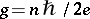has a natural topological interpretation as a connection in theprincipal bundle over the two-sphere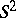with the first Chern number (the winding number)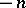(cf. Connections on a manifold; Principal fibre bundle; or [a3] for a review). In natural units, the potentialscan be written as one-forms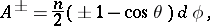and they are a connection one-form written in two charts covering. More precisely,,above are coordinates of the two-sphere. Then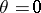is the north pole andis well-defined everywhere outside the north pole, for example on a chart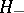covering the southern hemisphere including the equator (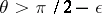). On the other hand,is the south pole, and thusis well-defined everywhere except the south pole, for example on a chart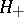covering the northern hemisphere including the equator (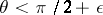). The intersection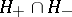is parametrized by the azimuthal angle. In order to combine this local system into a-principal bundle, onthe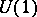-coordinate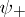overmust be related to the-coordinateoverby, with integer. This explains the appearance of Dirac's string singularity when the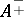are extended to, and the fact that it can be removed by a gauge transformation which requires Dirac's quantization condition. Thus, the trivial bundle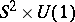admits no monopole (charge-monopole). The existence of a monopole indicates non-triviality of a corresponding principal bundle. The monopole of charge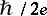is the connection in the Hopf fibration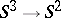, while the monopole of charge withcorresponds to the-bundle overwith the lens spaceas a total space (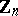is viewed inside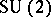as a subgroup ofth roots of the unit matrix) [a7].

The Dirac monopole is an example of an Abelian monopole, i.e., a solution of field equations of gauge theory with Abelian gauge group. Since the mid-1970{}s there has been a considerable interest in non-Abelian monopoles, in particular those related to thegauge theories. In pure mathematics this was triggered in particular by the appearance ofgauge theory in the classification of four-manifolds by S.K. Donaldson [a2]. However, in 1994, E. Witten [a8] showed that certain Abelian monopole equations motivated by the supersymmetric quantum field theory [a5], [a6] and known as the Seiberg–Witten equations, can be used to derive both the Donaldson invariants of four-manifolds as well as new ones (the Seiberg–Witten invariants; cf. also Four-dimensional manifold). It was soon noted [a4] that the Dirac gauge potentialwith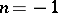provides a bosonic part of the simplest (not) solution to Seiberg–Witten equations. Witten's observation, as well as the appearance of magnetic monopoles in string theory, revived the interest in both monopoles and the reciprocity between electric and magnetic charges (electric-magnetic duality).

How to Cite This Entry:
Dirac monopole. Encyclopedia of Mathematics. URL: http://encyclopediaofmath.org/index.php?title=Dirac_monopole&oldid=13354
This article was adapted from an original article by T. Brzezinski (originator), which appeared in Encyclopedia of Mathematics - ISBN 1402006098. See original article